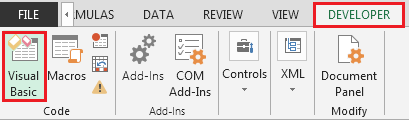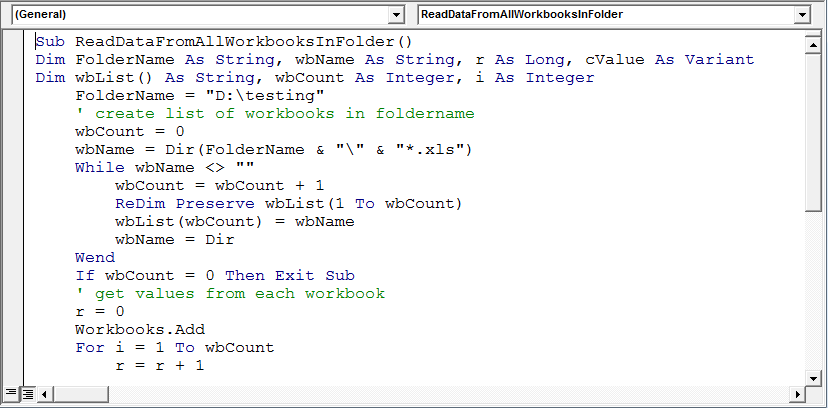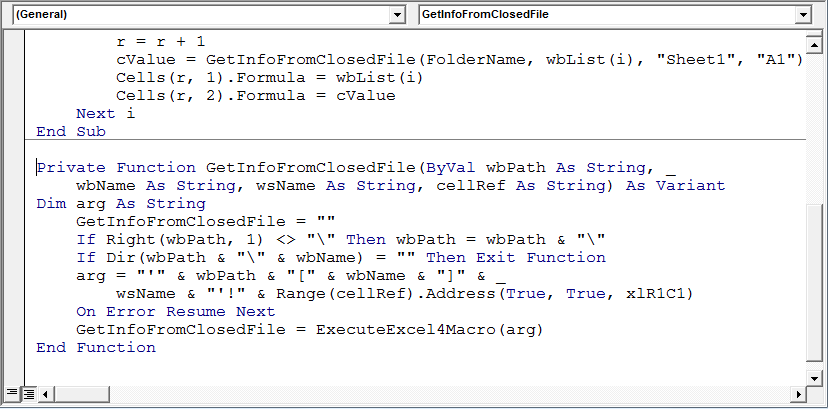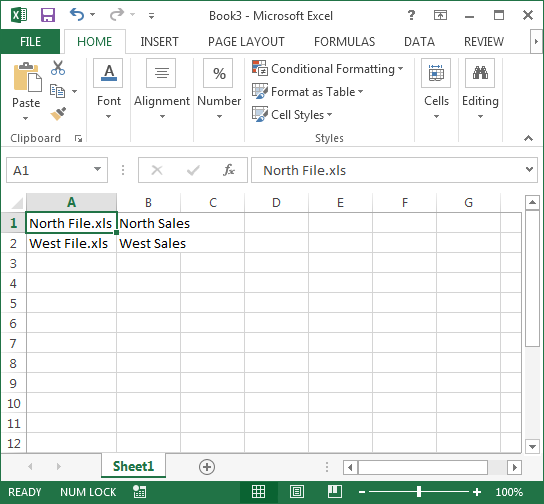# Read information from a closed workbook using VBA in Microsoft Excel

In Microsoft Excel, getting information from a closed workbook is one of the most time-saving ways to get accurate data using VBA code. In this article, we will learn how to read information from a closed workbook using VBA in Microsoft Excel.

If you are getting multiple files through email or any other source & you want to get information stored in a particular cell, then you should read this tutorial.

To understand this example, we need to create some sample files in a path which we will use in VBA code; here, we have saved couple of files (North & West files) in “D:\testing” path.

To extract data from all the excel files in a folder, we need to follow the below steps to launch VB editor:

• Click on Developer tab
• From Code group select Visual Basic• Copy the below code in the standard module

```Sub ReadDataFromAllWorkbooksInFolder()
Dim FolderName As String, wbName As String, r As Long, cValue As Variant
Dim wbList() As String, wbCount As Integer, i As Integer
FolderName = "D:\testing"
' create list of workbooks in foldername' --- Comment
wbCount = 0
wbName = Dir(FolderName & "\" & "*.xls")
While wbName <> ""
wbCount = wbCount + 1
ReDim Preserve wbList(1 To wbCount)
wbList(wbCount) = wbName
wbName = Dir
Wend
If wbCount = 0 Then Exit Sub
' get values from each workbook' --- Comment
r = 0
For i = 1 To wbCount
r = r + 1
cValue = GetInfoFromClosedFile(FolderName, wbList(i), "Sheet1", "A1")
Cells(r, 1).Formula = wbList(i)
Cells(r, 2).Formula = cValue
Next i
End Sub

Private Function GetInfoFromClosedFile(ByVal wbPath As String, _
wbName As String, wsName As String, cellRef As String) As Variant
Dim arg As String
GetInfoFromClosedFile = ""
If Right(wbPath, 1) <> "\" Then wbPath = wbPath & "\"
If Dir(wbPath & "\" & wbName) = "" Then Exit Function
arg = "'" & wbPath & "[" & wbName & "]" & _
wsName & "'!" & Range(cellRef).Address(True, True, xlR1C1)
On Error Resume Next
GetInfoFromClosedFile = ExecuteExcel4Macro(arg)
End Function
```We need to run the macro & following will be the snapshot of result:Conclusion: Using above code, we can get data from closed workbook from a specific cell.

Note: Cell A1 is used as an example; if you want to retrieve data from any other cell, then you simply need to mention that cell.We would love to hear from you, do let us know how we can improve, complement or innovate our work and make it better for you. Write us at info@exceltip.com

## Users are saying about us...

1.Usage of DIR is explained well.
wbName = Dir() also works fine.
Sub GetDataFromClosedWorkbook() is very useful.
Thanks.

2.dear sir, when i followed your article useing the method2, I got an error when it run at

With ThisWorkbook.Worksheets("xxxx")

It return "Out of array range index"

But when I replace it with
With ThisWorkbook.ActiveSheet

Then it ran without error, but no data can got load.

3.As an alternative, I use JetSQL to pull data from closed Workbooks very fast...

4.When the line Workboks.open is executed, a VBAProject(Filename) is added to the Project-VBAProject list.
Is there a way to supress this?
Thanks

5.How would I alter this to get A1 to A500?

6.Hi, I am wondering for code to read entire excel sheet data. I searched a lot but unfortunately did not get a code to read and print entire data from an excal sheet. Can any one help me ? Thanks in advance.

Yogi

7.Hi, I am wondering for code to read entire excel sheet data. I searched a lot but unfortunately did not get a code to read and print entire data from an excel sheet. Can any one help me ? Thanks in advance.

Yogi

8.Hi,
Your routine (if i can get it to work) to extract a single cell from every workbook in a folder will be perfect for me. Unfortunately every time i try and load it to run i get a Syntax error on this line;

wbName = Dir(FolderName & "\" & "*.xls") "" with the following comment also, Compile error: Expected end of statement

Appreciate any help/guidance you can give me 🙂
•```Dir(FolderName & "\" & "*.xls")